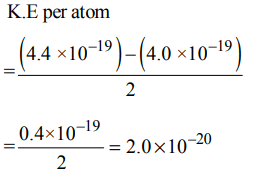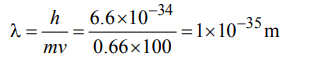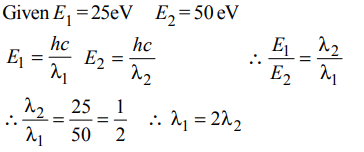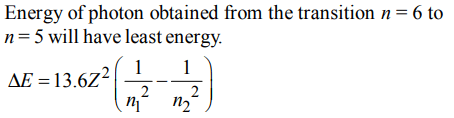## Atomic Structure Questions and Answers Part-14

1. The energy absorbed by each molecule $\left(A_{2}\right)$ of a substance is $4.4×10^{-19}j$    and bond energy per molecule is  $4.0×10^{-19}j$    . The kinetic energy of the molecule per atom will be:
a) $2.2×10^{-19}J$
b) $2.0×10^{-19}J$
c) $4.0×10^{-20}J$
d) $2.0×10^{-20}J$

Explanation:2. Maximum number of electrons in a subshell of an atom is determined by the following
a) $2\ell+1$
b) $4\ell-2$
c) $2n^{2}$
d) $4\ell+2$

Explanation: The number of sub shell is (2 l + 1). The maximum number of electrons in the sub shell is 2 (2 l + 1) = (4 l + 2)

3. Which of the following is not permissible arrangement of electrons in an atom?
a) n = 5, l = 3, m = 0, s = + 1/2
b) n = 3, l = 2, m = – 3, s = – 1/2
c) n = 3, l = 2, m = – 2, s = – 1/2
d) n = 4, l = 0, m = 0, s = – 1/2

Explanation: m = – l to +l, through zero thus for l = 2, values of m will be – 2, –1, 0, + 1, + 2
Therefore for l = 2, m cannot have the value –3.

4. A 0.66 kg ball is moving with a speed of 100 m/s. The associated wavelength will be $\left(h=6.6*10^{-34} Js\right)$    :
a) $1.0 * 10^{-32}m$
b) $6.6 * 10^{-32}m$
c) $6.6 * 10^{-34}m$
d) $1.0 * 10^{-35}m$

Explanation:5. The total number of atomic orbitals in fourth energy level of an atom is :
a) 8
b) 16
c) 32
d) 4

Explanation: Total no. of atomic orbitals in a shell = n2
Given n = 4; Hence number of atomic orbitals in 4th shell will be 16.

6. The energies  $E_{1} and E_{2}$  of two radiations are 25 eV and 50 eV, respectively. The relation between their wavelengths i.e., $\lambda_{1}$ and $\lambda_{2}$ will be :
a) $\lambda_{1}=\lambda_{2}$
b) $\lambda_{1}=2\lambda_{2}$
c) $\lambda_{1}=4\lambda_{2}$
d) $\lambda_{1}=\frac{1}{2}\lambda_{2}$

Explanation:7. If n = 6, the correct sequence for filling of electrons will be :
a) $ns\rightarrow\left(n-2\right)f\rightarrow\left(n-1\right)d\rightarrow np$
b) $ns\rightarrow\left(n-1\right)f\rightarrow\left(n-2\right)d\rightarrow np$
c) $ns\rightarrow\left(n-2\right)f\rightarrow np\rightarrow\left(n-1\right)d$
d) $ns\rightarrow np\rightarrow \left(n-1\right)d\rightarrow\left(n-2\right)f$

Explanation:8. According to the Bohr Theory, which of the following transitions in the hydrogen atom will give rise to the least energetic photon ?
a) n = 6 to n = 1
b) n = 5 to n = 4
c) n = 6 to n = 5
d) n = 5 to n = 3

Explanation:9. The value of Planck’s constant is $6.63 × 10^{-34} Js$   . The speed of light is $3 × 10^{17}$ nm $s^{-1}$  Which value is closest to the wavelength in nanometer of a quantum of light with frequency of $6 × 10^{15} s^{-1}$    ?
a) 25
b) 50
c) 75
d) 10